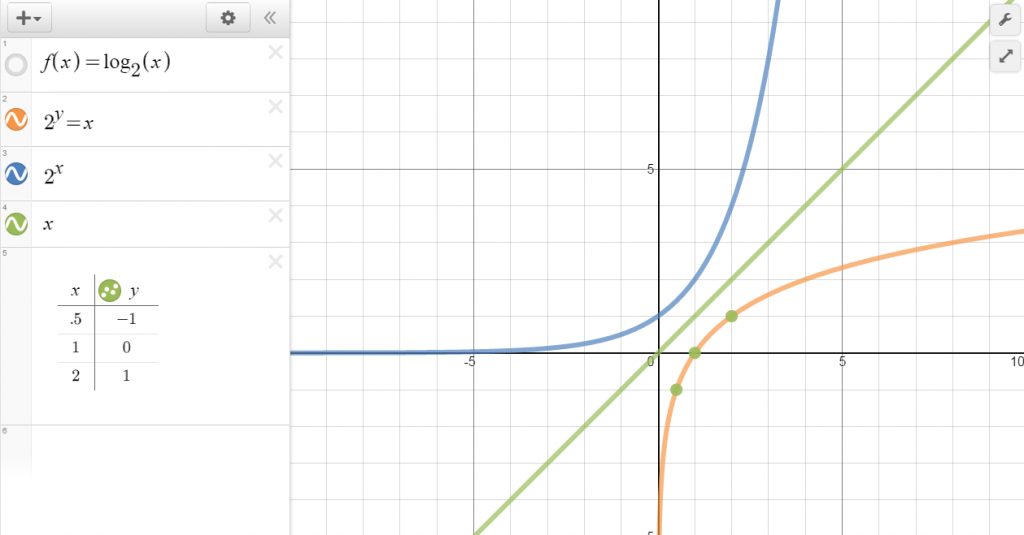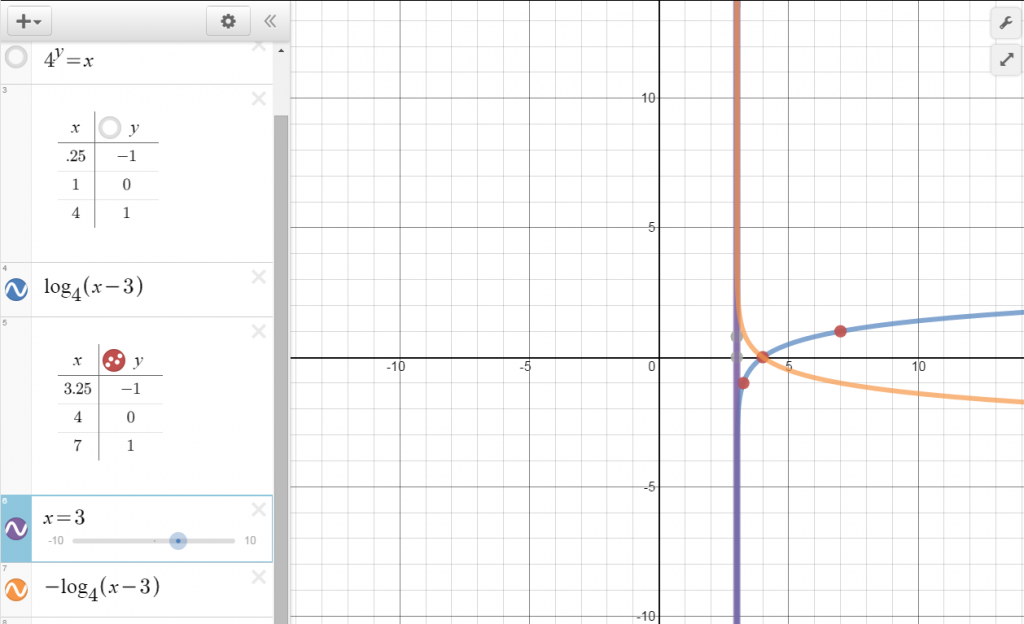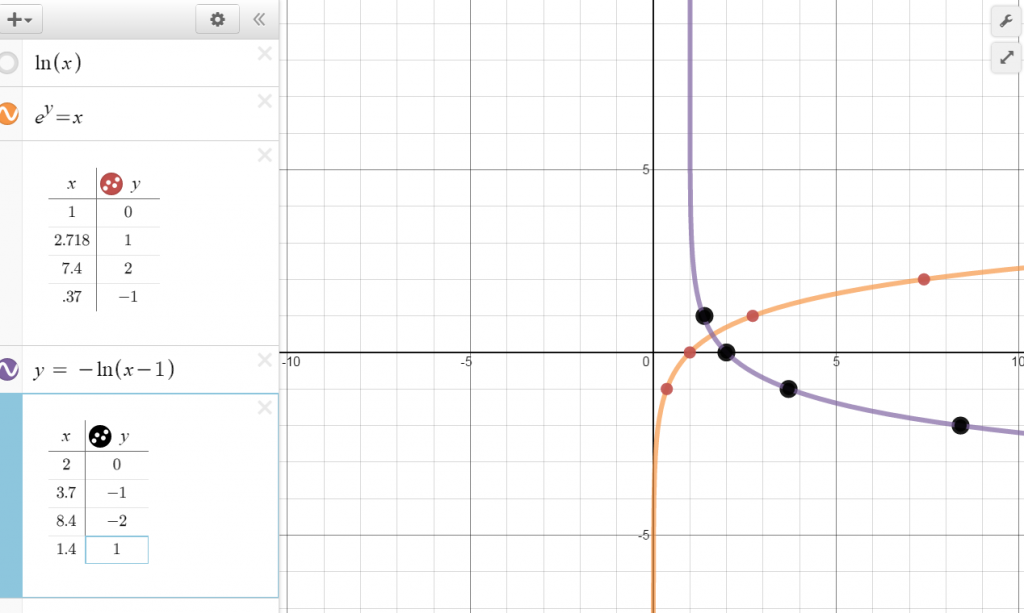# Logarithmic Functions

//Logarithmic Functions
Logarithmic Functions 2017-11-13T22:01:53+00:00

Just as addition is the inverse of subtraction and multiplication and division are inverses of one another, logarithms and exponents are inverse operations.

## Definition of a Logarithmic Function with Base a

A logarithmic function is defined as y = loga x if and only if ay = x

For x > 0 and a > 0, and a ≠1

That means the answer to a logarithmic equation is the exponent of an exponential equation.

How would we write the following in exponential form?

Log10 100 = 2

Log­3 81 = 4

28= 256

53 = 125

170 = 1

## Properties of Logarithms

loga 1 = ________ because

loga a = ________ because

log a ax = ________ because

If log a x = log a y then

log ­4 16 =

log 6 1 =

log 9 3 =

log 2 (-1) =

### Graph y = log 2 x

Start by writing it in exponential form.

2y = x

Now, plug in values for y.This should remind you of a function you have been introduced to earlier:

f(x) = 2x

How are the two related?

Graph y = log 4 (x – 3)

Remember your families of graphs? Start by graphing your parent function of y = log ­­4 x. Then shift it 3 to the right.## The Natural Log

e – Euler’s Number, Napier’s Constant, the Natural Base

e ≈ 2.718281

e is the base in the natural log noted as ln.

So ln = log­­e ≈ log 2.718­

Graph y = ln x

Graph y = -ln (x – 1)Because order of operations says to do what’s in parenthesis first, shift left one first, then reflect across the x-axis.

If your logarithmic function involves a reflection and a vertical shift like this function – y = -ln x + 2, first reflect over the x – axis, then shift up 2 because the multiplication of the -1 would take place before the addition of 2.

Solve log 12 (x – 3) = log 12 (2x + 5)

Precalculus with Limits – Larson & Hostetler p. 229-238